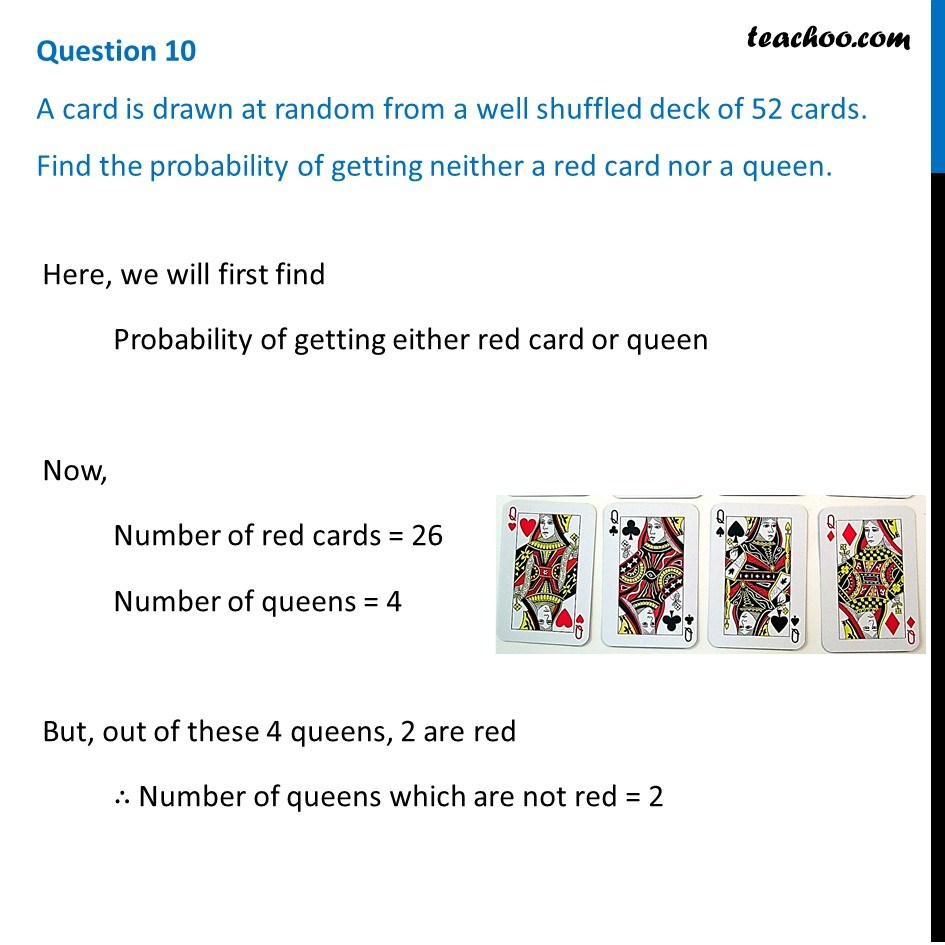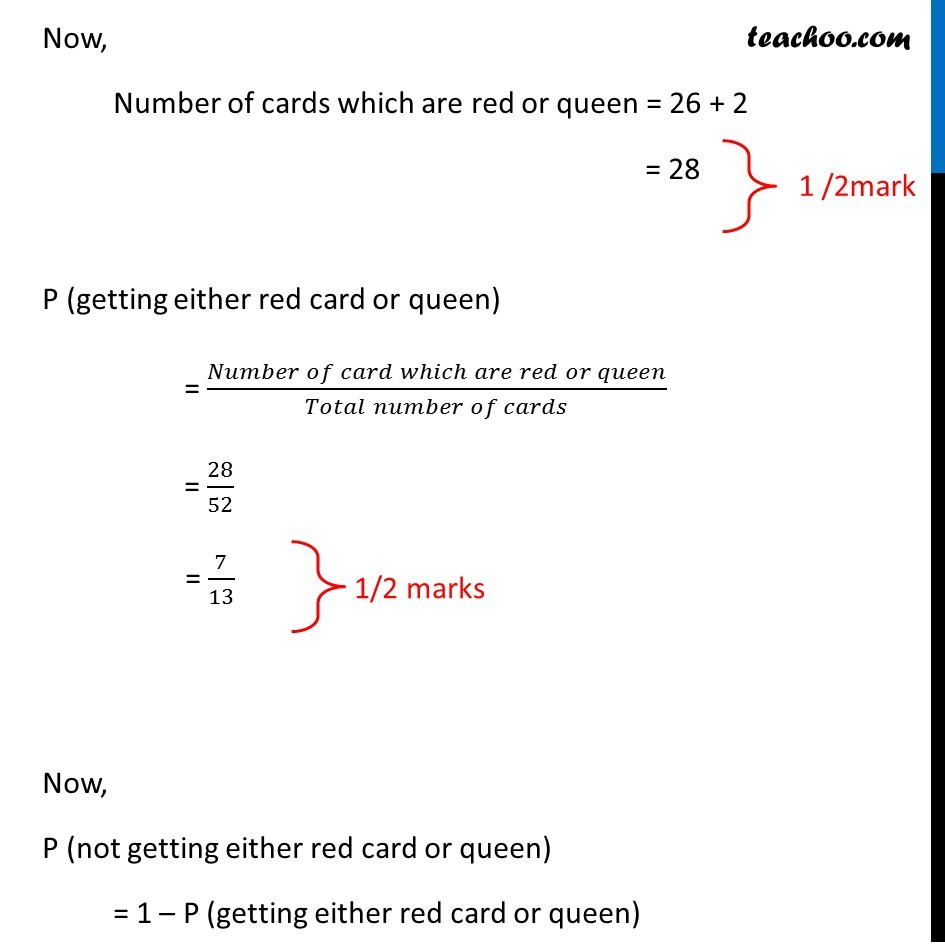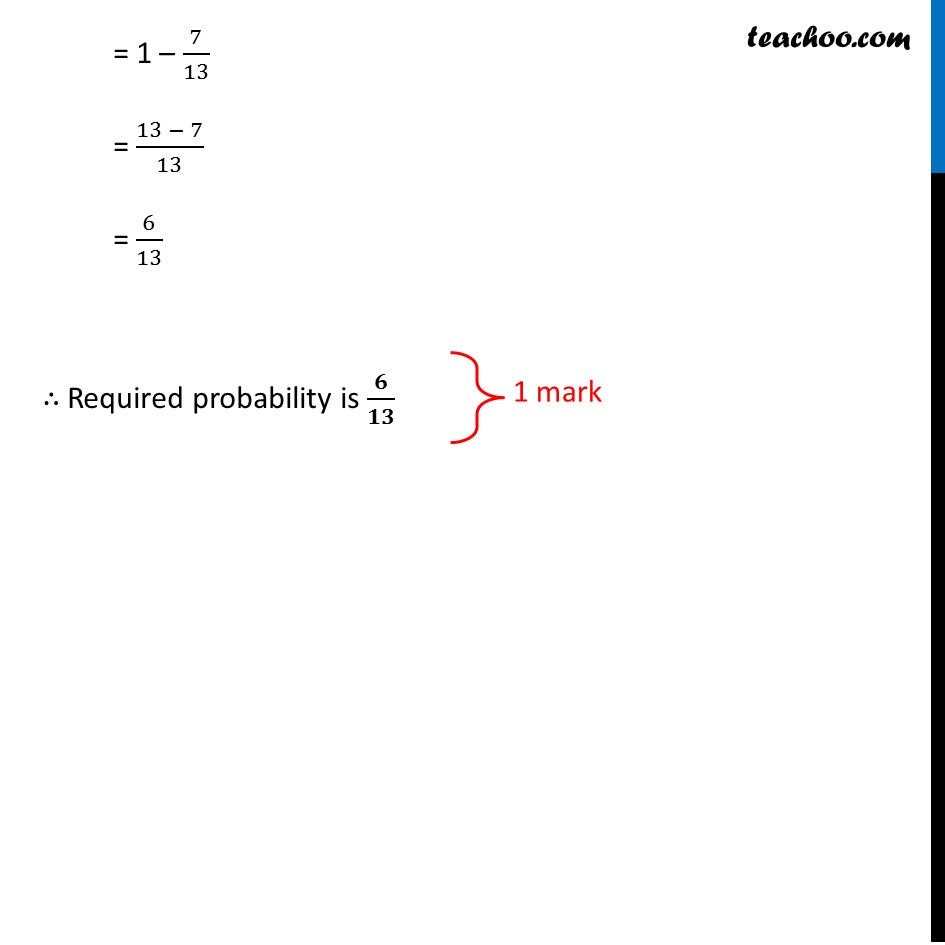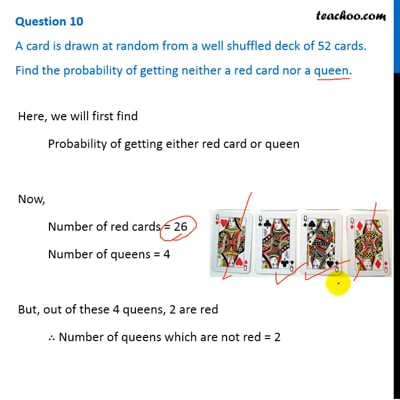CBSE Class 10 Sample Paper for 2019 Boards

Class 10
Solutions of Sample Papers for Class 10 Boards

Questi on 10

A card is drawn at random from a well shuffled deck of 52 cards. Find the probability of getting neither a red card nor a queen.This video is only available for Teachoo black users

### Transcript

Question 10 A card is drawn at random from a well shuffled deck of 52 cards. Find the probability of getting neither a red card nor a queen. Here, we will first find Probability of getting either red card or queen Now, Number of red cards = 26 Number of queens = 4 But, out of these 4 queens, 2 are red ∴ Number of queens which are not red = 2 Now, Number of cards which are red or queen = 26 + 2 = 28 P (getting either red card or queen) = (𝑁𝑢𝑚𝑏𝑒𝑟 𝑜𝑓 𝑐𝑎𝑟𝑑 𝑤ℎ𝑖𝑐ℎ 𝑎𝑟𝑒 𝑟𝑒𝑑 𝑜𝑟 𝑞𝑢𝑒𝑒𝑛)/(𝑇𝑜𝑡𝑎𝑙 𝑛𝑢𝑚𝑏𝑒𝑟 𝑜𝑓 𝑐𝑎𝑟𝑑𝑠) = 28/52 = 7/13 Now, P (not getting either red card or queen) = 1 – P (getting either red card or queen) = 1 – 7/13 = (13 − 7)/13 = 6/13 ∴ Required probability is 𝟔/𝟏𝟑